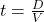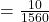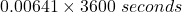Question

Two planes approach each other head-on. Each has a speed of and they spot each other when they are initially 10.0 km apart. How much time do the pilots have to take evasive action?

1.23.08 seconds

Explanation:

The computation of the time required is shown below

Relative Velocity,V is

= 780 – ( – 780)

= 1560 km/h

As it is moving in inverse or an opposite direction

And, the Distance, D = 10 km

So the time is= 0.00641 hours

== 23.08 seconds

By dividing the distance from the relative velocity we can get the time and the same is to be taken in the computation part